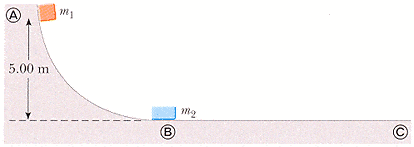# Momentum/Elastic Collision

Consider a frictionless track as shown in Figure P6.48. A block of mass m1 = 5.55 kg is released from A. It makes a head on elastic collision at B with a block of mass m2 = 11.0 kg that is initially at rest. Calculate the maximum height to which m1 rises after the collision.I used to .5mv^2 = mgh to get the velocity of the first block at the bottom. That was 9.9 m/s.

Then I did m1vi + m2vi = m1vf + m2vf => m1vi + 0 = 0 + m2vf to solve for the speed of the second block when the first block has gone back up the ramp and stopped. I then used the energy in the first equation and conservation of energy to solve for the final height.

KE = m1gh + .5m2v2f

But this gave me a height of 4.49 meters which WebAssign says is not correct. Thanks for any help. By the way, it's due at 8:30 EST tomorrow morning.

Last edited:

Chi Meson
Homework Helper
You're conservation of momentum equation is incorrect. Both blocks are moving after the collision, so $$m_1 v_1 = m_1 v_1 _f + m_2 v_2 _f$$

Since there are two unknowns, you need two equations. What else do you know to be true for elastic collisions?

I gotta log off, but here's a hint: KINETIC ENERGY.

and there's a really simple way of doing this that doesn't involve squared speeds.

Last edited:
You're conservation of momentum equation is incorrect. Both blocks are moving after the collision, so $$m_1 v_1 = m_1 v_1 _f + m_2 v_2 _f$$

Since there are two unknowns, you need two equations. What else do you know to be true for elastic collisions?

That energy is conserved. So I use that momentum equation and
KE = m1v1f^2 + 11v2f^2

Solve for v1f and I get 9.9 and -3.3. So I use -3.3 and plug that into a new KE = PE for the amount of energy that the first block has now. And now I'm getting within 10% of the answer so I rounded badly somewhere. Great........... Thanks for the help.

Chi Meson
Homework Helper
That energy is conserved. So I use that momentum equation and
KE = m1v1f^2 + 11v2f^2

Solve for v1f and I get 9.9 and -3.3. So I use -3.3 and plug that into a new KE = PE for the amount of energy that the first block has now. And now I'm getting within 10% of the answer so I rounded badly somewhere. Great........... Thanks for the help.

Yeah, it's not 3.3, it's 3.25. You've got 3 sigs here.

And the easier way I mentioned in the edit to my last post is:

the relative velocity between 2 bodies in a 1-D elastic collision stays constant. So: v1 - v2 = v2' - v1'

try it, it saves lots of time.

Ah cool. Thanks a lot.

Yeah, I did a quadratic and it was a pain.

I need to look up that equation and stuff cus I don't see off the bat how masses don't matter but ok.